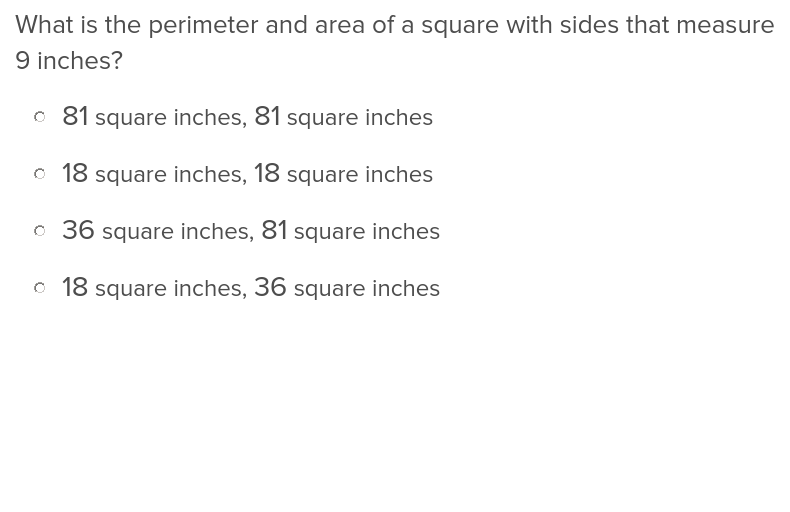Learning Library
Who we are
Guidance

# 3.MD.D.8 Worksheets, Workbooks, Lesson Plans, and Games

#### CCSS.MATH.CONTENT.3.MD.D.8

:
"Solve real world and mathematical problems involving perimeters of polygons, including finding the perimeter given the side lengths, finding an unknown side length, and exhibiting rectangles with the same perimeter and different areas or with the same area and different perimeters."

These worksheets can help students practice this Common Core State Standards skill.

## Worksheets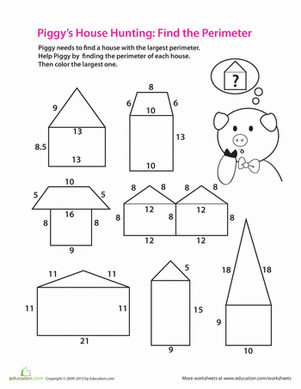Find the Perimeter
Worksheet
Find the Perimeter
In this 3rd grade math worksheet, your child will practice geometry and addition as she calculates the perimeter of each house to find the largest one.
Math
Worksheet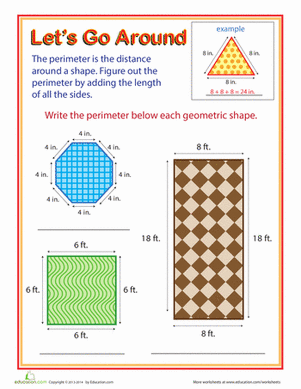Let's Go Around! Exploring Perimeter
Worksheet
Let's Go Around! Exploring Perimeter
Hunting for a worksheet to help your kid with geometry? This printable will help him find the perimeter of objects.
Math
Worksheet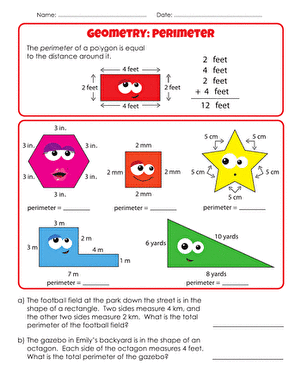Geometry Basics: Perimeter
Worksheet
Geometry Basics: Perimeter
Practice perimeter with your third grader with this nifty worksheet.
Math
Worksheet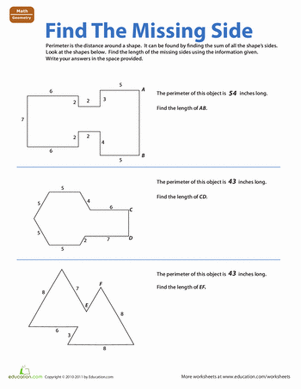Find the Missing Side
Worksheet
Find the Missing Side
Get your child acquainted with the concept of perimeter with this geometry worksheet
Math
Worksheet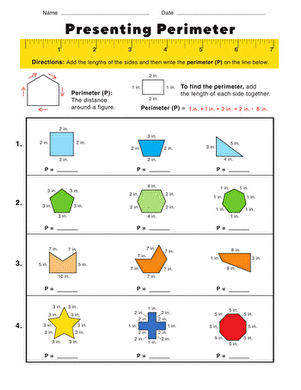Presenting Perimeter
Worksheet
Presenting Perimeter
Kids learn how to calculate the perimeter of a shape, and practice their addition facts while they're at it.
Math
Worksheet

## Lesson Plans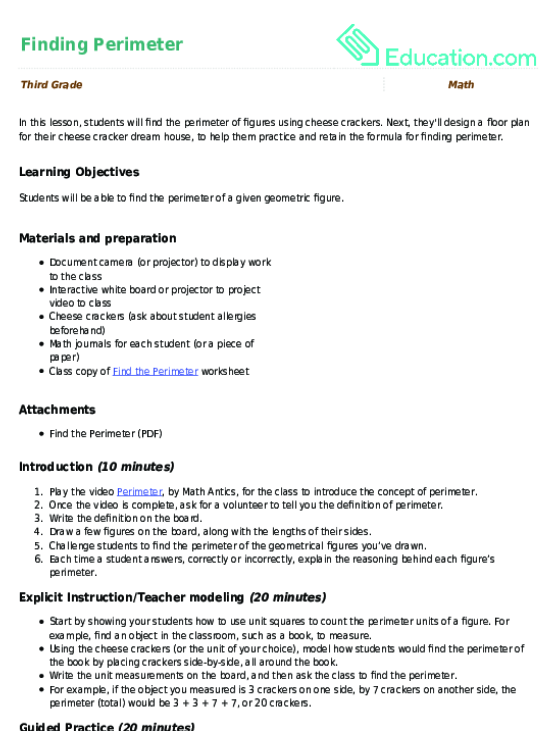Finding Perimeter
Lesson plan
Finding Perimeter
In this lesson, students will find the perimeter of figures using cheese crackers. Next, they'll design a floor plan for their cheese cracker dream house, to help them practice and retain the formula for finding perimeter.
Math
Lesson plan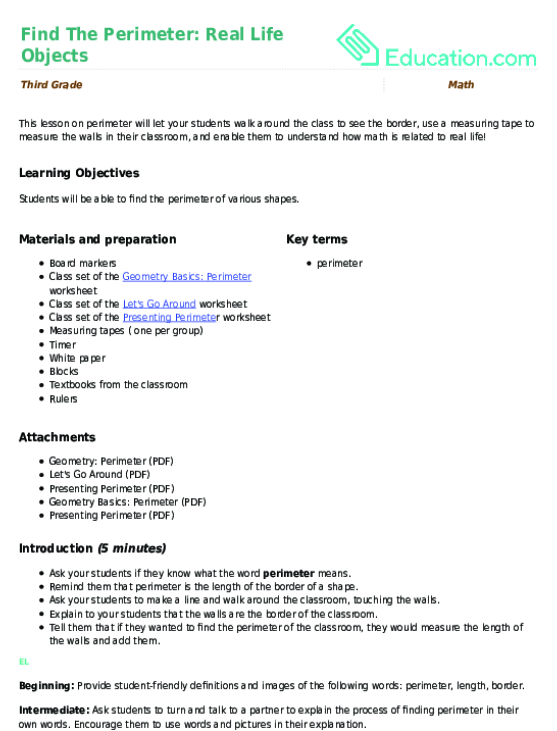Find The Perimeter: Real Life Objects
Lesson plan
Find The Perimeter: Real Life Objects
This lesson on perimeter will let your students walk around the class to see the border, use a measuring tape to measure the walls in their classroom, and enable them to understand how math is related to real life!
Math
Lesson plan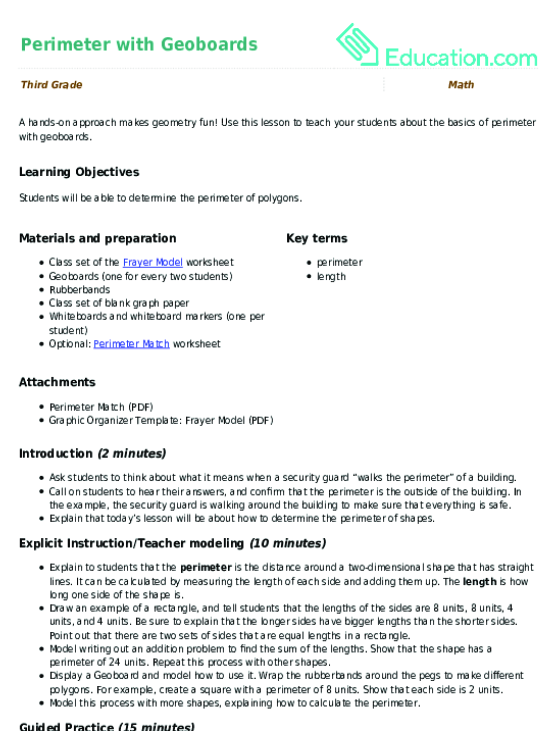Perimeter with Geoboards
Lesson plan
Perimeter with Geoboards
A hands-on approach makes geometry fun! Use this lesson to teach your students about the basics of perimeter with geoboards.
Math
Lesson plan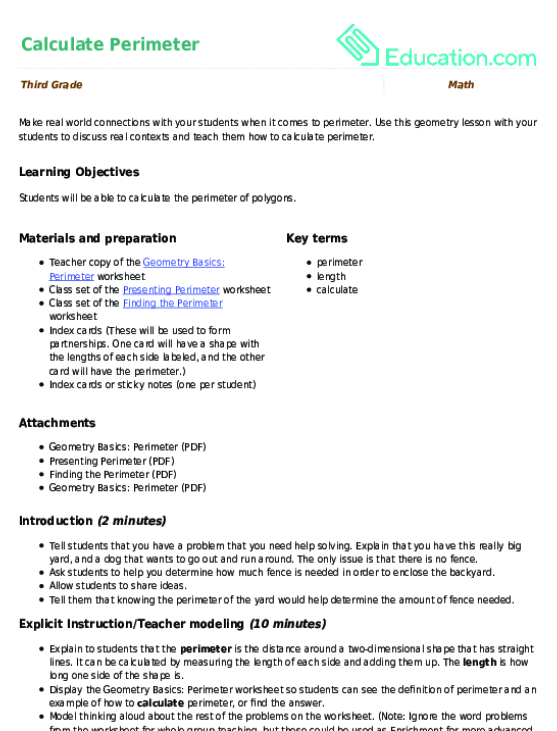Calculate Perimeter
Lesson plan
Calculate Perimeter
Make real world connections with your students when it comes to perimeter. Use this geometry lesson with your students to discuss real contexts and teach them how to calculate perimeter.
Math
Lesson planPerimeter of Polygons
Lesson plan
Perimeter of Polygons
Finding the perimeter of a polygon is a simple process with addition! Use this lesson to teach your students how to find the perimeter of polygons.
Math
Lesson planPolygon Perimeters with Tantalizing Tangrams!
Lesson plan
Polygon Perimeters with Tantalizing Tangrams!
Teach your students to find the perimeter of polygons using real world examples. They will make polygon pictures using tangrams and practice finding the perimeters of the polygons in their masterpieces.
Math
Lesson plan

## Workbooks

No workbooks found for this common core node.

## Games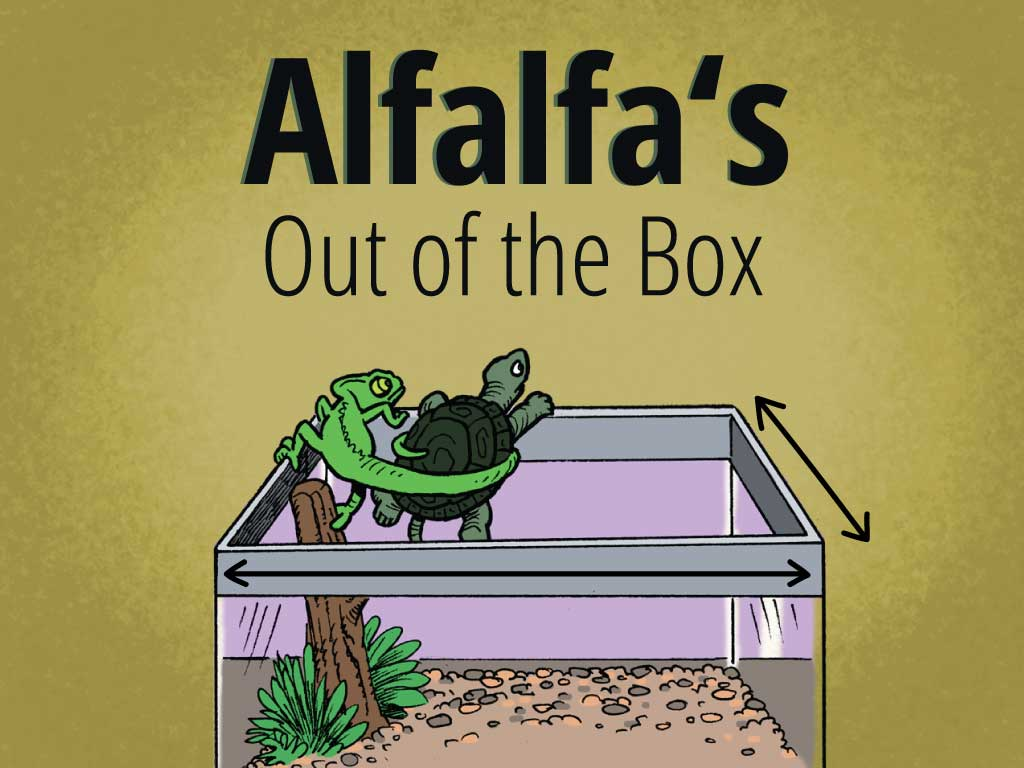Alfalfa's Out of the Box: Perimeter, Area, and Addition
Game
Alfalfa's Out of the Box: Perimeter, Area, and Addition
Kids help Alfalfa the turtle and Old Harold navigate their way into the backyard in this sweet math story.
Math
Game

## Exercises